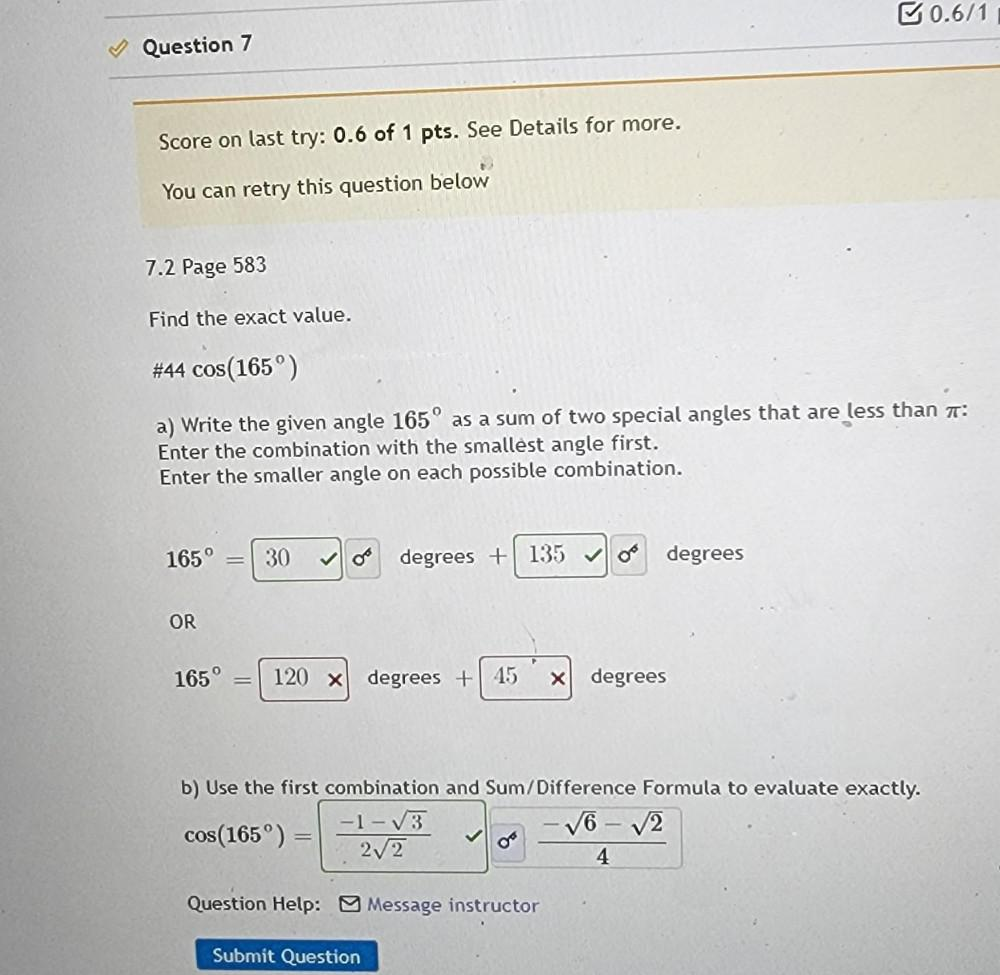Question:

# Write the given angle 165° as a sum of two special angles that are less than 7: Enter the combination with the smallest angle first. Enter the smaller angle on each possible combination. 165° = degreeWrite the given angle 165° as a sum of two special angles that are less than 7: Enter the combination with the smallest angle first. Enter the smaller angle on each possible combination. 165° = degrees +degrees OR 165° = degrees + degrees b) Use the first combination and Sum/Difference Formula to evaluate exactly. cos(165°)=